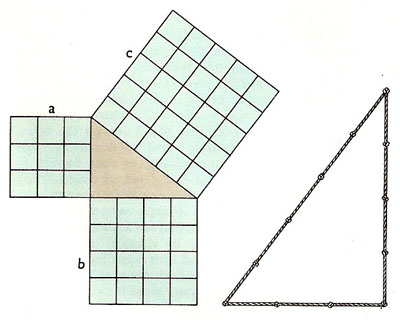A# Pythagoras' theoremPythagoras' theorem demonstrated in the case of the 3, 5, 5 triangle (one having sides in the ratio 3:4:5). It can be seen by inspection that in this case a2 + b2 = c2. Other right-angled triangles, the ratios of whose sides can be expressed using only small integers are 5, 12, 13 and 8, 15, 17 triangles. Right: The ancient method of laying out a right angle using a knotted rope was known and used long before the time of Pythagoras.

Pythagoras' theorem states that the square of the length of the hypotenuse (the longest side) of a right triangle is the sum of the squares of the lengths of the other two sides. This is usually expressed as a2 + b2 = c2.